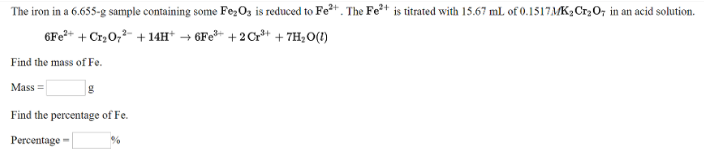# Problem: The iron in a 6.655-g sample containing some Fe 2O3 is reduced to Fe2+. The Fe2+ is titrated with 15.67 mL of 0.1517M K2Cr2O7 in an acid solution. 6Fe2+ + Cr2O72- + 14H+ → 6Fe3+ + 2Cr3+ + 7H2O(l) Find the mass of Fe. Find the percentage of Fe.

###### FREE Expert Solution
79% (236 ratings)View Complete Written Solution
###### Problem Details

The iron in a 6.655-g sample containing some Fe 2O3 is reduced to Fe2+. The Fe2+ is titrated with 15.67 mL of 0.1517M K2Cr2O7 in an acid solution.

6Fe2+ + Cr2O72- + 14H+ → 6Fe3+ + 2Cr3+ + 7H2O(l)

Find the mass of Fe.

Find the percentage of Fe.